# Leetcode 15–3Sum

This article will cover and explain a solution to the Leetcode problem 3Sum. I recommend you first solve Two Sum and/or Two Sum 2 prior to this. With that being said, strap in, tie those shoes, and let’s get into it!

# Problem Overview

Description:

Given an integer array nums, return all the triplets `[nums[i], nums[j], nums[k]]` such that `i != j`, `i != k`, and `j != k`, and `nums[i] + nums[j] + nums[k] == 0`.

Notice that the solution set must not contain duplicate triplets.

Constraints:

`0 <= nums.length <= 3000`

`-10^5 <= nums[i] <= 10^5`

This is pretty clear with exception to a few edge cases:

• What do we return if `nums.length < 3` ? (Examples later on show to return `[]` )
• Assuming duplicate here implies order doesn’t matter for equality; meaning, 2 solution arrays are unique if they differ by at least 1 element.

# Example

`Input: nums = [-1,0,1,2,-1,-4]`
`Output: [[-1, 2, -1],[-1 , 0, 1]]`

This doesn’t require much explaining. There were 2 combinations of 3 numbers that when summed equaled 0.

# Strategy, Concepts, and Solution

Alright, so we need unique pairs of 3 numbers which sum to 0, and those numbers we choose must be from 3 different indices in the array. Naturally, you might think of doing three nested loops and brute forcing, which would look something like below: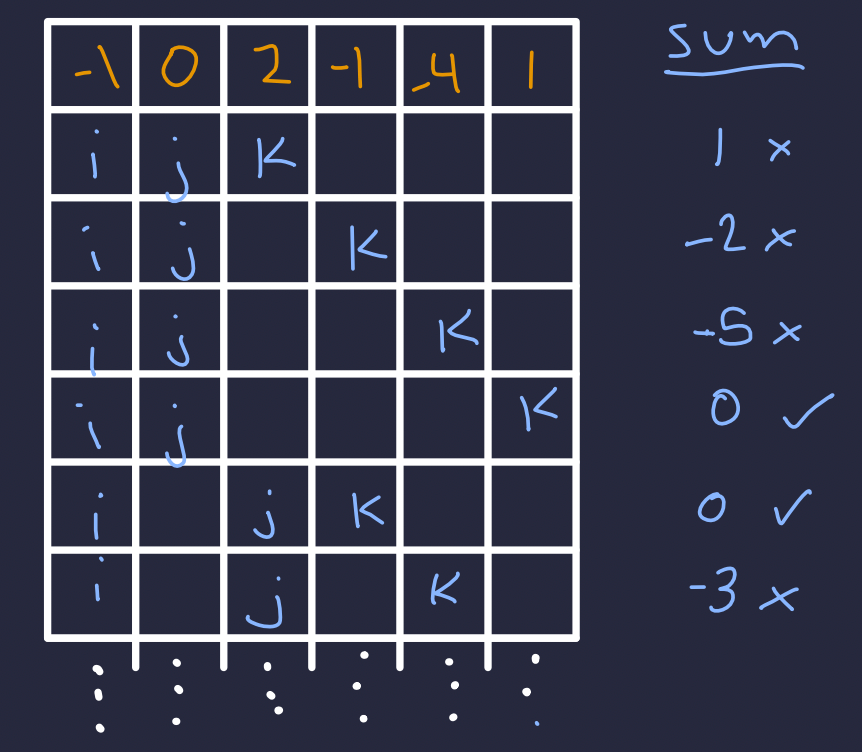The outer for loop advances i, the middle j, and the inner k.

But, this has`O(N^3)` and we can do MUCH better. How? First, let’s sort the input array. After sorting, we get an array in which we can use a 2 pointer approach to find 2 values that sum to a target. As an example, a 2 sum problem is shown here (Review Two Sum 2):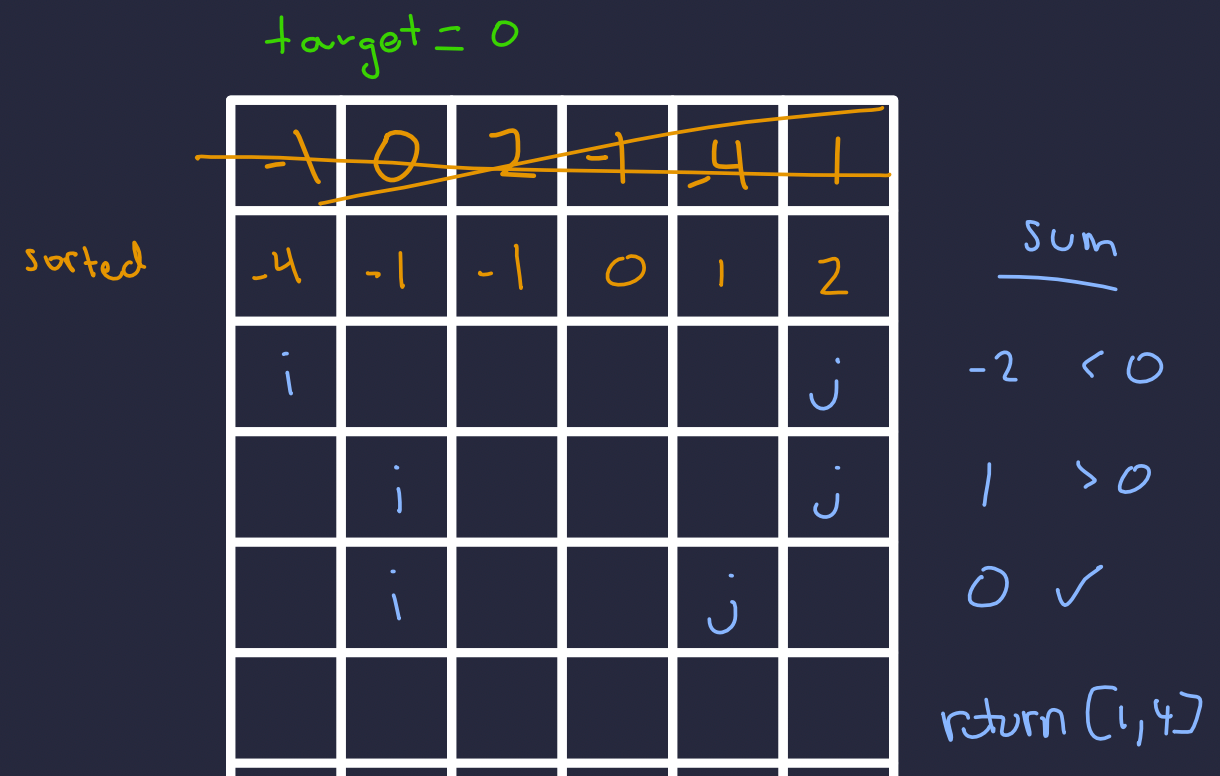If the sum is less than target, increment i (left) otherwise decrement j (right) until we find our target. Note, this is NOT the optimal binary search solution for 2Sum, but use here for a specific reason explained below.

So, how is this useful for 3Sum? Well, we can break down the 3 element sum into a 2 element sum!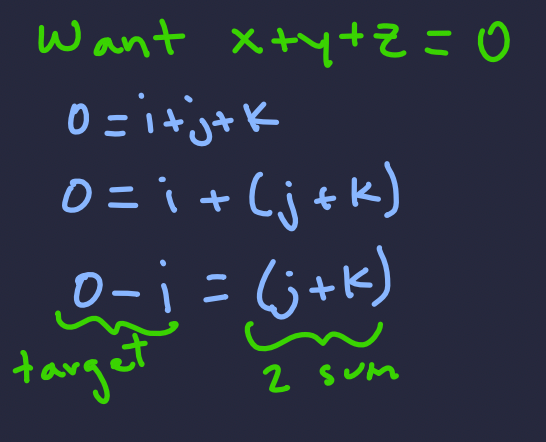We can transform our 3 sum problem into a 2 sum subproblem!

This 3 sum problem turns out to just be a 2 sum problem with an extra step! From here, we can see that 1 loop will advance `i`and a nested loop will solve the two sum problem given the current`i`(as shown above). Below we walk through an illustration of this: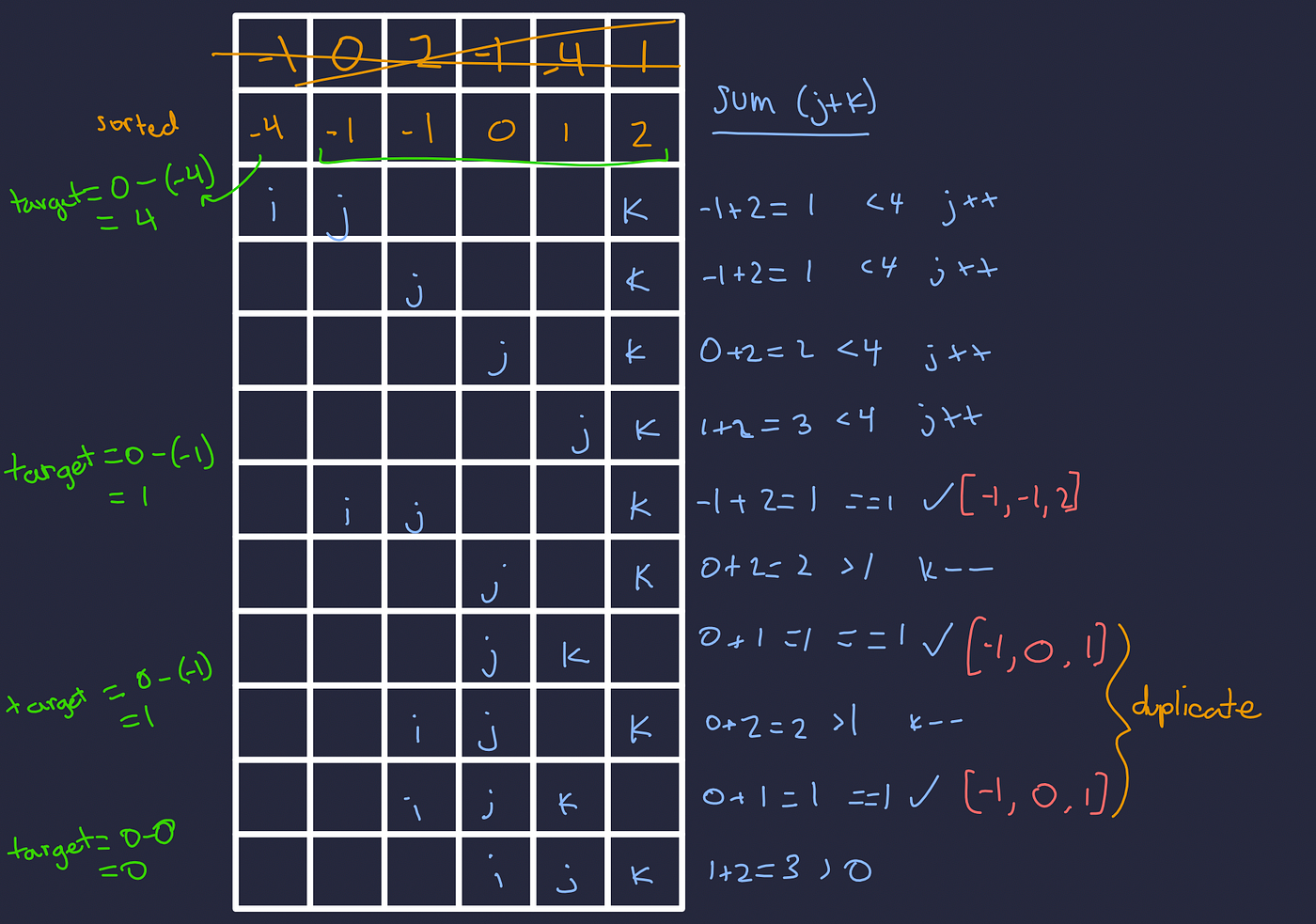Example problem order of operations when breaking it down to 2Sum problems. Note, we sum the element values at indices j and k, not the literal index.

Let’s unpack this. In green we have each new target when `i`advances through the loop. Orange is our input and callout to a problem we encountered. Blue are the steps that are done each iteration. Lastly, red highlights successfully found tuples.

So, we see that given position `i`, we simply need to solve 2 sum where the input array spans `array[i+1:len(array)]`and `target=0-i` ! But wait, this doesn’t handle the requirement that we need unique tuples. The orange ‘duplicate’ calls this out above. What should we do? Simply check if the value we advanced to was the same as the previous, if so skip it.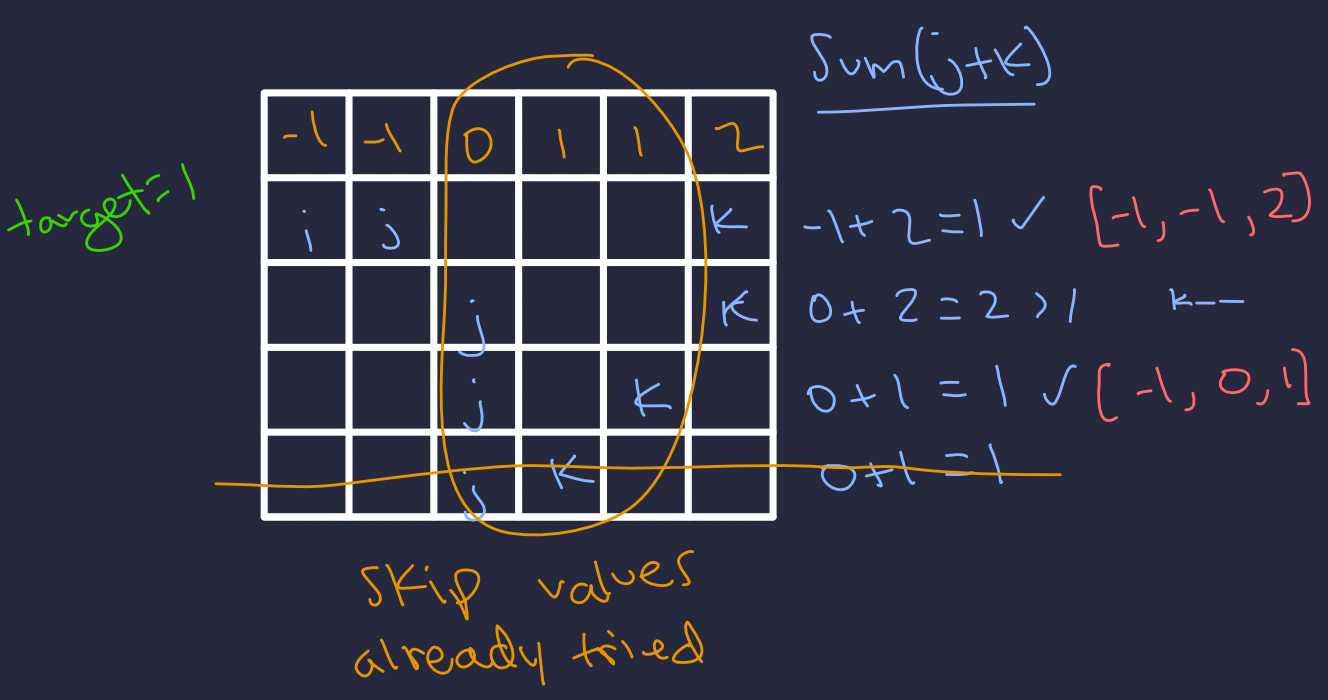Skipping duplicates in this way applies to all index pointers i, j, k.

Now that our duplicates issue is handled, we’re just about ready to get coding. First, let’s evaluate the time complexity: `O(nlogn + n^2) := O(n^2)` . This is due to the sort being `O(nlogn)` and our 2 loops being`O(n^2)` .

Now, that we’ve got an idea of what to do and some time complexity analysis done, let’s go ahead and code it!

I hope you enjoyed the article :)!

--

--## Thomas Higginson

Software Engineer working on Cognitive EW capabilities, and human that enjoys making smiles. Welcome.